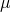Feb 152011

What is the probability that your investments will fall short of a particular investment goal?  How is this probability affected by uncertainty about the equity risk premium?

In this post, I plan to look at a simple method for estimating the probability that a risky investment will fall short of a particular return goal, and I’ll then extend this analysis to allow for uncertainty about the equity risk premium.

This analysis is a very simplified example of the concept presented by Lubos Pastor and Robert F. Stambaugh in their paper “Are Stocks Really Less Volatile in the Long Run?”.

For starters, if we assume stock prices follow a random walk, then the probability of a risky investment underperforming a risk free investment, such as a T-bill, is given by this equation:Where:
r = continuously compounded (log return) t-bill rate= continuously compounded (log) equity return= annual standard deviation of equity returns
T = number of years